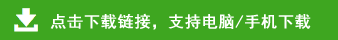# 三年级数学下册期中试卷及答案共五套

三年级数学下册期中试卷及答案共五套部分内容预览

1、直接写得数（8分）
120×3=           540×2=          5×800=           205×3=
400×7=           16×5=           1200÷4=           30÷3=
2、用竖式计算（2×3=6分）
906×8=                  460×3=                  504×6=
3、脱式计算（3×4=12分）
306×3×4      900－25×5        (620＋180)×8        72×5÷4

55÷5  △  55×5        56+6　△　56×6          78×4  △  78×0
8千克 △ 7900克        2000千克 △ 20吨        27×2  △  72×2

1、填合适的单位。
（1）一盒牙膏重约35（     ）。
（2）我的体重大约是25（     ）。
（3）一节火车皮大约能装货物（     ）。
（4）一盒订书针重50（     ）。
2、4kg=（     ）g        15t=（     ）kg     8000kg=（     ）t
3、（    ）÷ 4 = 100        （    ）+497=1341       3×（    ）= 42
4、按规律填数
（1）1，3，9，27，81，（    ），（    ）
（2）1，4，9，16，（    ），（    ），49，64
（3）90，81，72，（    ），（    ），（    ），（    ），36，27